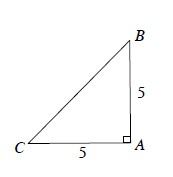### Home > PC > Chapter 3 > Lesson 3.1.3 > Problem3-48

3-48.

$\triangle CAB$ is an isosceles triangle with $\overline { C A } = \overline { B A }$.1. Find the exact length of $\overline{B C}$. Express your answer in simplified radical form.

Use the Pythagorean Theorem.

2. Write the sine ratio for $\angle C$. Reduce the fraction.

$\text{sin}C=\frac{5}{5\sqrt2} = \frac{1}{\sqrt2}$

3. Rationalize the denominator of the fraction you got in part (b) by multiplying the numerator and denominator by $\sqrt { 2 }$.

$\frac{1}{\sqrt2} = \left(\frac{1}{\sqrt2}\right)\left(\frac{\sqrt2}{\sqrt2}\right) = \frac{\sqrt2}{\sqrt{4}} = \frac{\sqrt2}{2}$